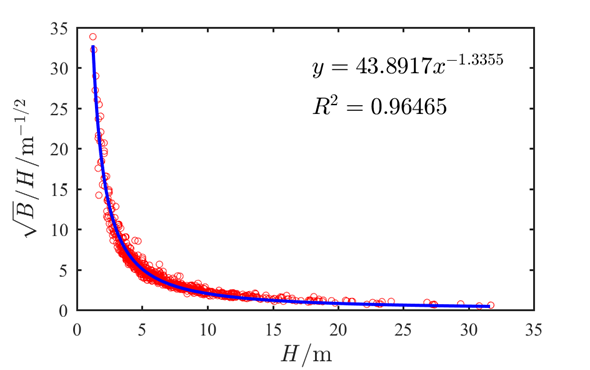# [mtalab学习记录03]matlab中输入LaTeX公式

2021/8/20 18:17:47 浏览：

### 文章目录

• 前言
• 一、LaTeX公式输入
• 1.1 LaTeX公式输入的通用格式
• 1.2 LaTeX常用符号表示
• 1.3 LaTeX公式输入常用命令
• 二、应用案例
• 2.1 基础案例
• 2.2 进阶案例

# 一、LaTeX公式输入

## 1.1 LaTeX公式输入的通用格式

 %格式一：'$表达式或值$'
%        一个公式只占一行当公式高度超过设定字符高度时会缩小公式中的字符
%格式二：'$$表达式或值$$'
%        一个公式能占多行公式中的每个字符都为设定的字符高度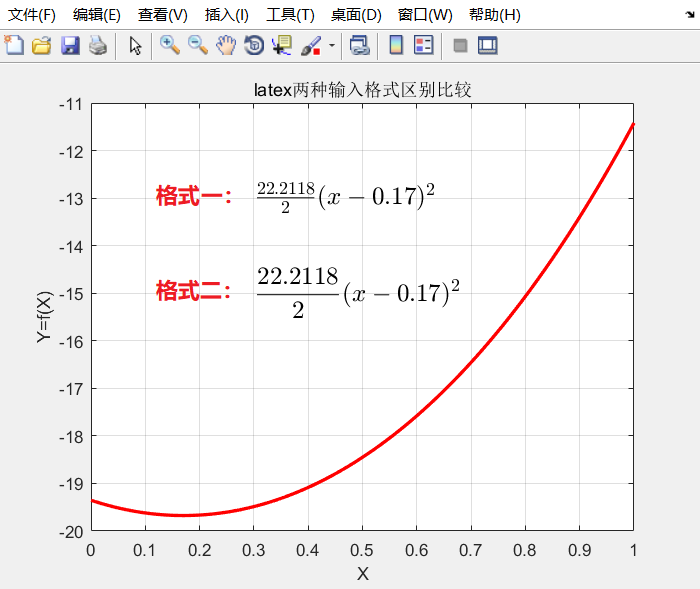## 1.2 LaTeX常用符号表示

LaTeX输入数学符号时一般直接输入以下的字符，如要输入α直接在matlab中输入\alpha即可。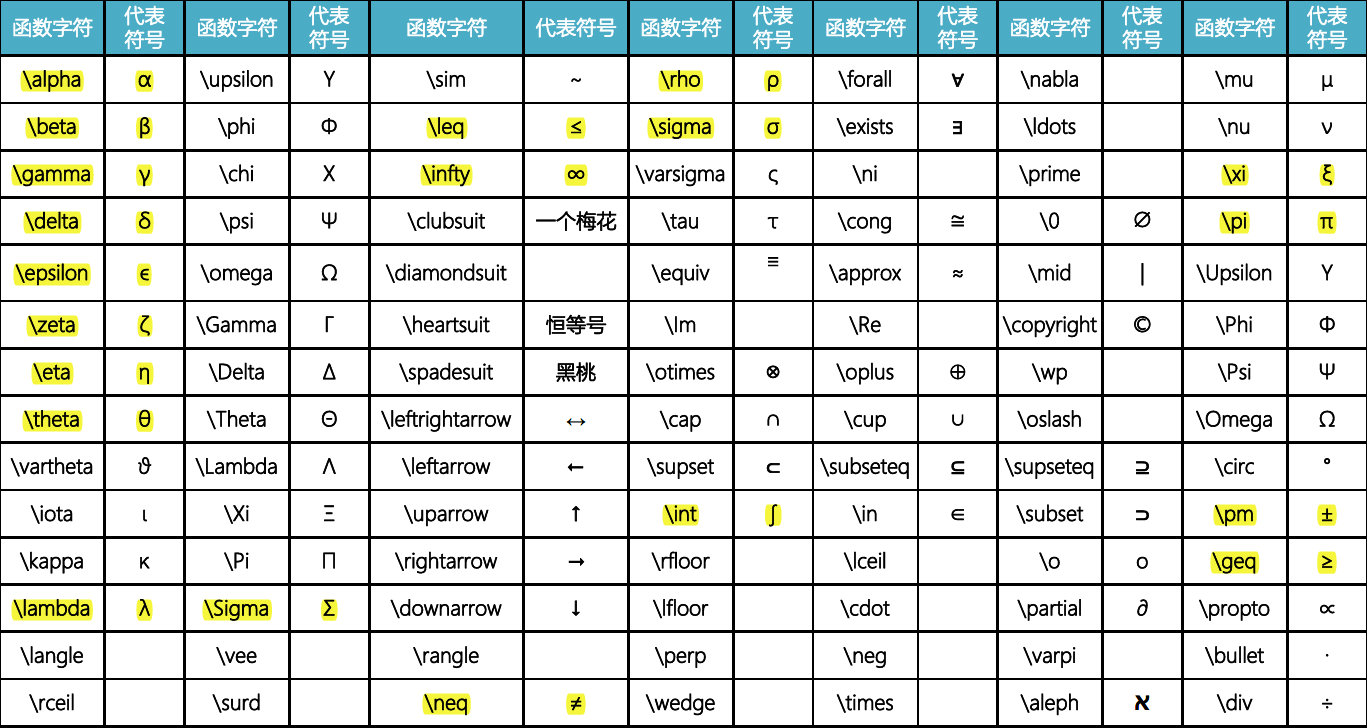## 1.3 LaTeX公式输入常用命令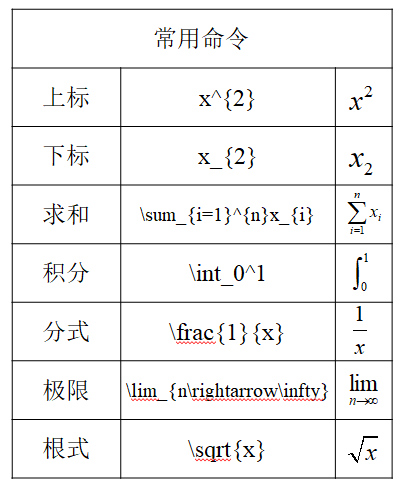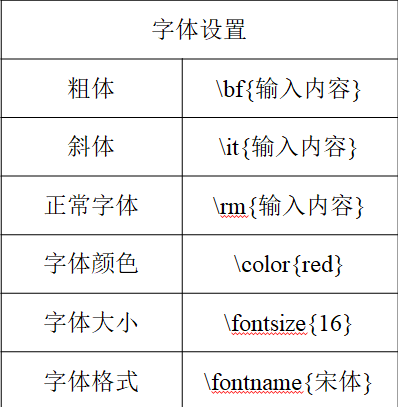# 二、应用案例

## 2.1 基础案例

clear;clc
x=0:pi/100:2*pi;
y1=x.*sin(x);
y2=(2*x.^2-5*x)/6;
plot(x,y1,'r-','linewidth',2)
hold on;
plot(x,y2,'b--','linewidth',2)
hold off;grid on;
xlabel('X');ylabel('Y');

text(2,2.5,['$$y=xsin(x)$$'],'interpreter','latex','fontsize',15,'color','red');
%2,2.5为指定输入文本的位置
%interpreter为输入公式采用的方式，此处采用LaTeX输入故应选择'latex'
text(3,5,['$$y=\frac{2x^{2}-5x}{6}$$'],'interpreter','latex','fontsize',15,'color','blue');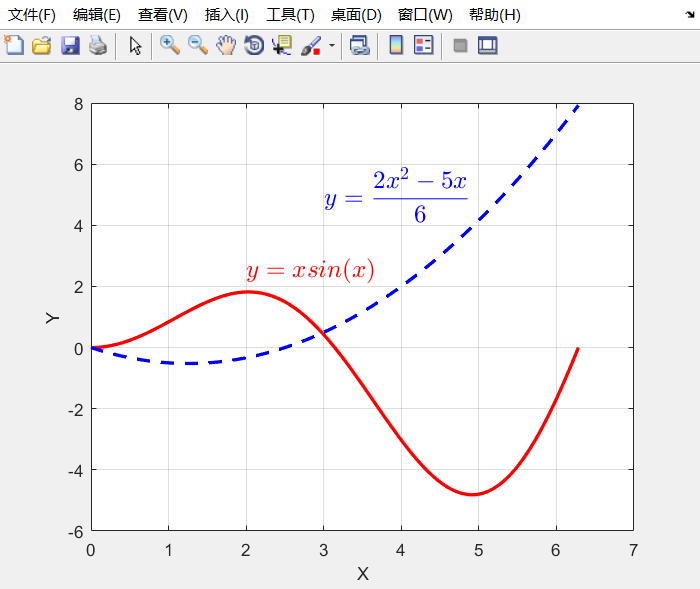## 2.2 进阶案例

clear；clc
%% 数据拟合
filename='D:\个人文件夹\软件学习\matlab学习\数据拟合练习.xlsx';
x=num(:,1);
y=num(:,2);
f=fittype('a*x^b','independent','x');   %定义函数
[model,gof]=fit(x,y,f);                 %拟合函数，model为系数结果，gof为拟合结果检验
result={'河相系数α',model.a;'河相系数β',model.b;'确定系数',gof.rsquare};
xlswrite(filename,result,1,'A742:B744');

%% 拟合结果可视化
figure(1)
set(gcf,'unit','centimeters','position',[10,10,8,5])
xp=min(x):0.1:max(x);
yp=model(xp);
plot(x,y,'ro','markersize',2.5,'linewidth',0.7);
hold on;
plot(xp,yp,'-b','linewidth',1.2);
hold off;
set(gca,'LineWidth',0.7,'FontSize',7,'FontName','Times New Roman');
xlabel('$\it{H}/\rm{m}$','interpreter','latex','fontsize',9,'fontname','times new roman'); %x轴标签输入公式，单位需要设置为正体，公式变量设置为斜体
ylabel('$\it{\sqrt{B}/H}/\rm{m^{-1/2}}$','interpreter','latex','fontsize',9,'fontname','Times New Roman');
% txt={['$\alpha=$',num2str(model.a)],['$\beta=$',num2str(model.b)],['$R^2=$',num2str(gof.rsquare)]};
txt={['$','y=',num2str(model.a),'x','^{' num2str(model.b) '}$'],['$','R^{2}=',num2str(gof.rsquare),'$']};%含参公式输入，公式中有参数应当如何输入
text([18 18],[30 25],txt,'interpreter','latex','fontsize',9);